DOWN SOUTH.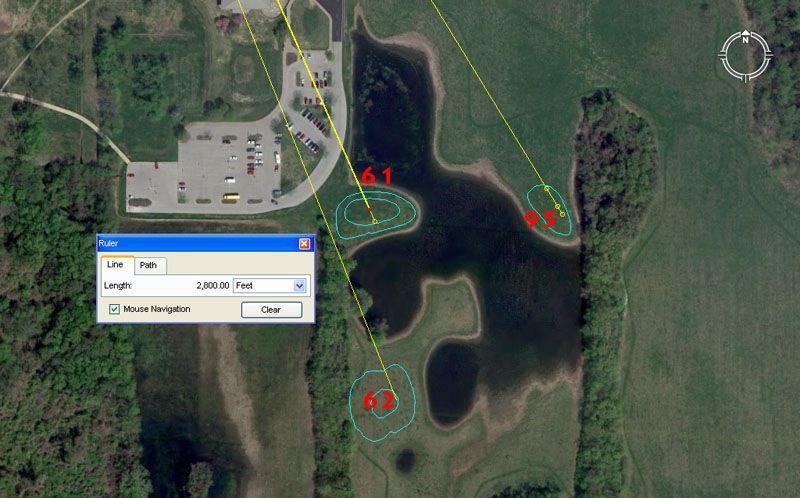Mounds 61, 62 and an out of sequence mound 95 sit around one of the flooded "borrow-pits" from which earth was taken to build the mounds.

Mound 61 sits 2800' out from the hub position on Monk's Mound @ 156.25-degrees. A second reading would have been 2835'.

A mathematical progression based upon 7 generates values used in calendar calculations or navigation, including 2800. A second tutorial that would have been included is 2835'. The Khafre Pyramid of Egypt has a base perimeter value of 2835' or 708.75' per side. The sum of 2835-days would equate to 8 lunar years of 354.375-days each.

A mathematical progression based upon 1.5625 will generate many useful values including 2 X 1.5625 = 3.125 (used as a form of PI in certain types of ancient calculations). The ancient English league @ 16500' was 3.125-miles. Also, the diameter of the Earth @ 7920-miles X 3.125 = 24750-miles (the equatorial circumference of the Earth under the "11" family of numbers, navigational system).

Mound 62 sits 3240' out @ an azimuth angle of 158.4-degrees.

The value 3240, used in a mathematical progression, generates many useful values for readings within the equatorial circumference of the Earth, the Precession of the Equinoxes and the lunar nutation cycle. For example, the sum of 324-years would equate to 1/80th of the 25920-year cycle of Precession and 324-days would be 1/21st part of the 6804-day lunar nutation cycle.

The degree angle codes the diameter of the Earth, of which 1584-miles would be 1/5th part.

Mound 95 sits 3000' out @ 148.5-degrees, Other distance readings would have included 2953.125' & 3024'.

Various readings which hover close to 3000' would have been taught at this mound, including the merits of 2953.125' (lunar) and 3024' (half of 1-minute of equatorial arc).

The angle of 148.5-degrees is navigational and the sum of 1485' equates to 1/4th of the 5940' Scottish mile.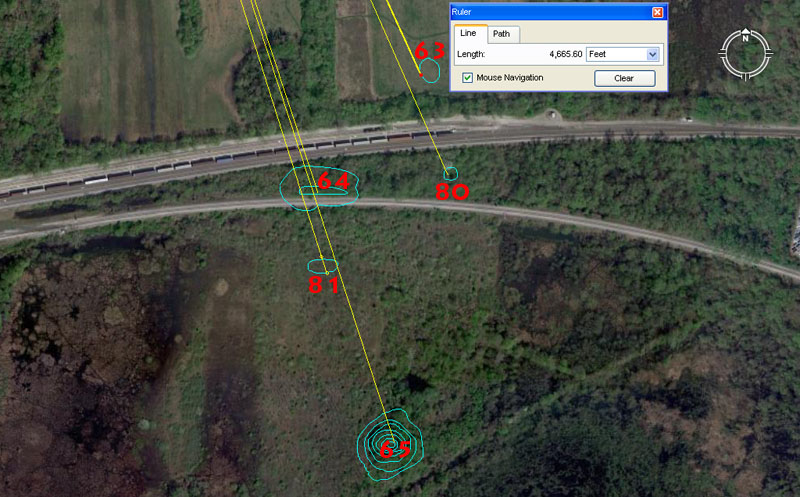Mounds 63, 64 & 65 sit within a cluster that includes mounds 80 & 81. The location is beyond the railway tracks in scrub (regenerating forest) country SSE of the park.

Mound 63 seems to carry a dynamic distance code of 4665.6' @ 155.52-degrees and both of these values are, essentially, a part of the same mathematical progression that relates to the equatorial circumference of the Earth. At Stonehenge, a marker on the Avenue sits a coded distance of 233.28' from site centre to indicate a circle 466.56' in diameter. The sum of 46656' would be 1/2800th of the 130636800' (24883.2-Greek mile) equatorial circumference. Similarly, the sum of 1555.2-miles = 1/16th of the 24883.2-mile equatorial circumference.

These numbers recur constantly in ancient weights and volumes. For example:

THE SUMERIAN-BABYLONIAN ROYAL STANDARD.

This slightly heavier standard was for payments to the royal treasury and some Babylonian weights of this type bear the inscription, "of the King".

 BABYLONIAN ROYAL SINGLE STANDARD. 1 Talent……… 466560 grains, equals: 60 Mina……… @ 7776 grains, or: 3600 Shekel….. @ 129.6 grains. BABYLONIAN ROYAL DOUBLE STANDARD. 1 Talent………933120 grains, equals: 60 Mina…….. @ 15552 grains, or: 3600 Shekel… @ 259.2 grains.

Mound 80 sits 5062.5' out @ 156.25-degrees. The distance figure is lunar coding and the sum of 50.625-days X 7 = 354.375-days or 1 lunar year.

The angle coding is the same as that of mound 61.

Mound 64 sits 4950' out @ 161.8034-degrees.

A distance of 495-miles would be 1/16th of the 7920-mile diameter of the Earth and 4950-miles would be 1/5th of the 24750-mile (of 5280') equatorial. circumference.

The degree angle is, of course, coding the PHI ratio X 100.

Mound 81 sits 5250' (1 Greek mile) from the hub position atop Monk's Mound @ 162.5-degrees. Other length readings onto this mound would have included 5184' (navigation, Precession) and 5200' (calendar).

The 1.625 value had some usage within calendar year calculations and is a division of 13, 26 & 52 (as in months, bi-weeks and weeks in a year).

Mound 65 sits 5940' out @ 162-degrees. This distance is 1-Scottish mile, which was 1-furlong or furrowlong greater that the English mile of 5280'. The sum of 5940' would be 1/22000th of the 24750-mile (130680000') equatorial circumference.

The 162-degrees azimuth angle is coding "rounded PHI" (1.62) which was one of the most used values of antiquity. The Great Pyramid @ 756' (9072") of length is 5600 rounded PHI inches of 1.62" each. The 6804-day lunar nutation cycle is 42 periods of 162-days. The sum of 162' would be 1/2240th of 1-degree of equatorial arc (362880') under the 24883.2 Greek mile system.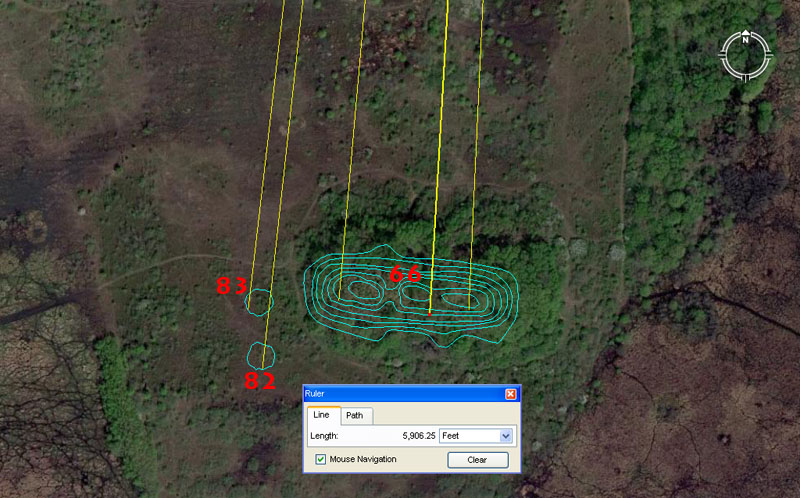Again, this cluster of mounds sitting almost due south of Monk's Mound hub are numbered out of sequence. The most substantial of the batch is mound 66.

Mound 66 sits a coded distance of 5906.25' from Monk's Mound hub and, because of its elongated configuration, carried several angle codes over its length. An eastwards angle code was 182.625-degrees (the number of days between equinoxes or half of 365.25 ... the number of days in a solar year). More centrally it sat 183.33333-degrees from the Monk's Mound hub and this value is a third of 550. Further west a return angle of 5-degrees works well.

The distance code is very important and is coding directly off the Khafre Pyramid of Egypt (Egypt's pyramid of the Moon). This is how one locates the lunar numbers mathematically and recognises them, by first going back to the Giza Plateau Bureau of Standards: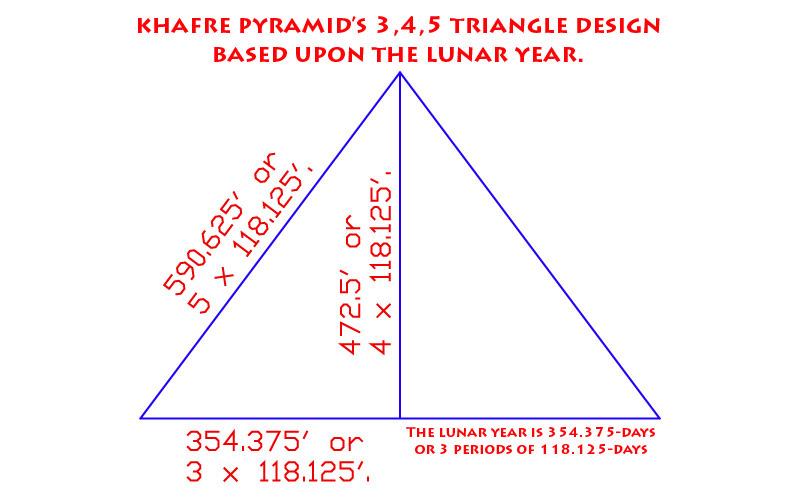The Khafre Pyramid of the Giza Plateau is 15/16ths the base length of the Great Pyramid. Therefore: 756' ÷ 16 = 47.25' X 15 = 708.75'. Half of the Khafre Pyramid's base length is 354.375' and the length of the lunar year (about 11-days shorter than the solar year) is 354.375-days.

1. Whereas the Great Pyramid, in its base length and slope angle (51.84-degrees), accentuates the mathematical principles related to the size of the Earth and how to grid reference the Earth for successful navigation, the Khafre Pyramid is all about the Moon.

2. The Khafre Pyramid has a design slope angle of 53.13010235-degrees and this angle occurs naturally in all 3-4-5 triangles. Therefore, half the base length is 354.375' (lunar year coding), the vertical height was designed to be 472.5' and the length of the sloping face was designed to be 590.625'.

3. With each base length of the pyramid equating to 708.75', a full circumnavigation was 2835' or 34020". This value gave mnemonic reference to the 6804-day lunar nutation cycle, the half value of which was 3402-days.

Mound 82 sits 6048' out @ 6.25-degrees return to Monk's Mound. This distance is, of course, 1-minute of equatorial arc under the "6&7" navigational system. The distance would also have been read as 6050' for a tutorial about 1-minute of arc under the "11" navigational system.

The return angle of 6.25-degrees is navigational coding and 6.25-miles would be 1/11th of 1-degree of arc (68.75-miles) under the "11" navigational system.

Mound 83 sits 5940' out @ 186.624-degrees. The distance is 1 ancient Scottish mile. The degree angle is navigational coding and 186624' would be 1/700th of the 24883.2 Greek mile equatorial circumference.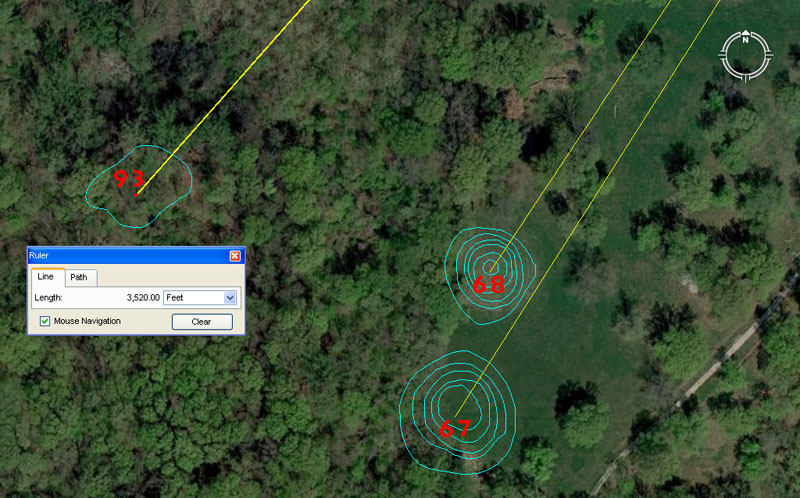Again, mound 93 sits out of numbering sequence but closeby mounds 67 & 68 to the SSW of Monk's Mound.

Mound 67 sits 3300' from the hub position atop Monk's Mound @ 33.6-degrees return. This length is 600 ancient fathoms, 50 chains (66') or 5 furlongs-furrowlongs (660'). It is also 1/5th of a league (16500').

The angle reading is also navigational coding and a mathematical progression based upon 336 will generate very exploitable divisions within the 24883.2 Greek mile circumference. The sum of 336' is 1/18th of 1-minute of arc (6048'). The ancient Irish mile @ 6720' is 3360' X 2.

Mound 68 sits 3500' out @ 32.4-degrees return. This distance is 2/3rds of a Greek mile. It is also 1/10th of an ancient Swedish Mil of 35000 English feet of 12". The ancient Swedish Mil was 6000 famn (fathoms) of 70" each (5.83333'). The Roman foot was 11.664" and their pace was 58.32", nevertheless, the evidence would suggest that these increments were limited to volume measures and lunar calculations. It's apparent that the Roman overland foot was the very simple to use 11.66666" (112/3rds) and that their overland pace or fathom was 58.333333" (581/3rd) in line with the ancient Swedish system.

The return angle of 32.4-degrees is a multi-use value and a mathematical progression based upon 324 generates numbers used in navigation, lunar cycle and Precession of the Equinoxes divisions and calculations. There would be 21 periods of 324-days in the 6804-day lunar nutation cycle and 80 periods of 324-years in the 25920-year duration of Precession.

Mound 93 sits 3520' out @ 41.472-degrees return. The distance is 640 ancient English fathoms of 5.5'. The sum of 352' would be 1/15th of a mile of 5280' and 3520' equates to2/3rds of a mile.

The degree angle is a dynamic value used in a mathematical progression that relates to the equatorial size of the Earth and the sum of 4147.2-miles is 1/6th of the 24883.2-mile equatorial circumference.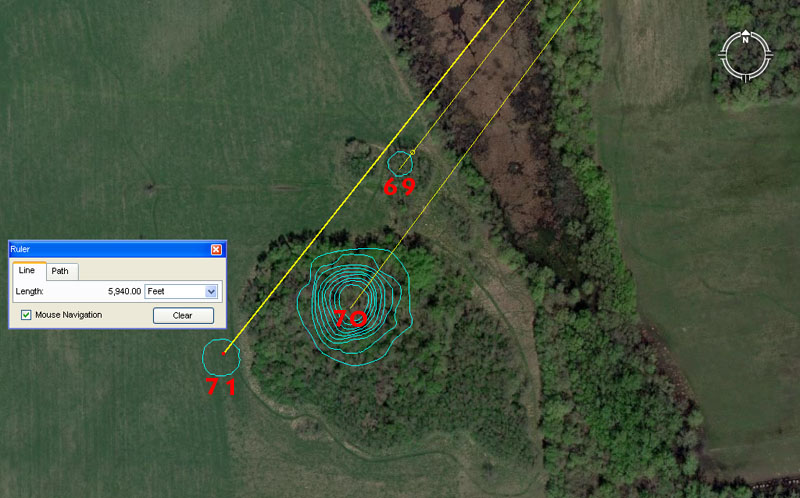Mounds 69, 70 and 71 sit a mile or so away to the SW of Monk's Mound.

Mound 69 sits exactly 1-mile (5280') out to its NE edge @ 217.8-degrees azimuth (37.8-degrees return to Monk's Mound). More centrally, the distance onto the remains of mound 69 is 5333.3333' (53331/3rd).

Yet again the 5280' increment is recorded into the position of a mound outer marker, just as with mound 3 of the Cahokia group. The more central distance is 5333.3333' and a mathematical progression, based up this value, generates 16000, 32000, 64000 increases and 5333.3333 is simply 1/3rd of 16000.

The 37.8-degrees return angle to Monk's Mound is in homage to the value 378 and the Great Pyramid @ 756' per side is 378' X 2. The sum of 378' would be 1/16th of 1-minute of equatorial arc.

Mound 70 sits 5670' out from the hub position atop Monk's Mound @ 36.666666-degrees (362/3rds). The distance is strong lunar coding and 567-days is 1/12th of the 6804-day lunar nutation cycle. The value was also used in navigational calculations and the sum of 5670' would be 1/64th of 1-degree of equatorial arc under the 24883.2 Greek mile circumference.

The return angle @ 36.66666-degrees is simply 1/3rd of 110. The sum of 36.66666' would equate to 1/144th of a 5280' mile. This kind of incremental value was essential to know when doing positional plotting at sea. For example:

If one uses Greek miles in sea legs of travel, the 5250' converts to a circumference of 16500' (1 English league) when 5250' is multiplied by PI @ 22/7ths. This means that the circumference generated from a 5250' diameter is in an "11" family of numbers and each degree of arc is 45.8333333' or 550". Therefore, if one chooses to divide the 16500' circuit up into half, quarter, eighth, sixteenth and thirty-second parts, etc., to get the primary and secondary points of the compass, then an increment of 366.6666' will divide the circle into 45 parts. Each 366.6666' will then equate to 8-degrees of arc or 550" X 8.

This kind of mental manipulation of, what is in reality, whole numbers and fractions, is exactly what the navigator had to be very adept at doing when calculating how far one had traveled in a straight line sea leg before a course change. Making one's way across the vast featureless ocean depended upon both the wind's direction and force, and voyages were made in a series of tacks on the breeze, zigzagging across a leyline towards a destination. Oftimes those angles of tack, back and forth, were quite severe. but the navigator had to stay constantly aware of exactly where the ship sat in the ocean at all times. Sea legs were completed to a whole value of, say, 5 Greek miles or 7 Greek miles (whatever was practicable and achievable without getting too far off course) before a new heading back across the leyline. The length of a leg (in whole Greek miles) was then used as a "diameter" on a plotting board, slate or papyrus map roll and turned into a calibrated "circle". Because the very experienced navigator, knew the boat speed, distance traveled and angle, he was able to accurately plot the latest completed sea leg information in perfect scale, then work out the exact degree angle (using the calibrated circle generated) back to the point of departure and onwards to the destination.

All of the positional plotting maths and increments used in sea legs are encoded into the Cahokia mound's landscape. For those of us who learnt our "times-tables" at elementary school by rote and repetition, that exercise pales into insignificance compared to what was happening amongst the mounds. This is where students came to learn the "times-table from hell", which included many strings of mathematical progressions. This school was only for the "brightest and the best" or most gifted children. Dunces and dullards or foot-dragging slackers had no place here and would not survive the grueling demands of the school ... in developing mental skills that allowed one to do complex calculations instantaneously in one's head ...or memorise many star positions and just how much the rise & set changed seasonally around the horizon...or how to use surveying devices to read angles accurately ...or how to toughen up to be equal with and endure the rigours and demands of the open sea, etc., etc.

Mound 71 sits 5940' out @ a return angle of 38.4-degrees.

Again, yet another mound encoded to sit 1 Scottish mile from the hub position atop Monk's Mound.

The 38.4 return degree angle is both lunar and navigational coding simultaneously and the value 384 is simply 96 X 4. The slightly elliptical Stonehenge site is 384' wide from North to South, but 378' wide from East to West (with the Avenue jutting out a bit further). Amongst the many uses for the number 384 is the fact that the inch value around the full perimeter of the Khafre Pyramid of Egypt (708.75' per side .... 2835' for 4 sides or 30420") X 3840 = 130636800, which, in feet, equates to the 24883.2 Greek mile circumference of the Earth.

Alternatively, the inch value around the full perimeter of Great Pyramid (756' per side .... 3024' for 4-sides or 36288") X 3600 = 130636800.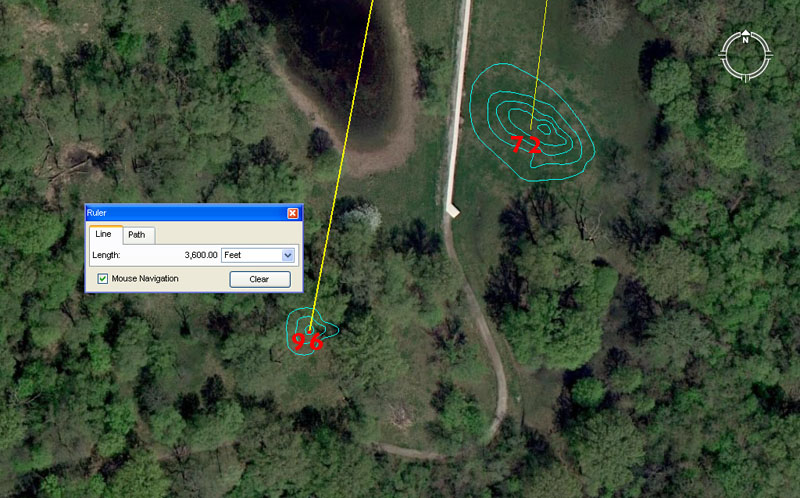Mound 72 sits 3333.33333' from the Monk's Mound hub at a return angle of 7.2-degrees. The length of 3360 would also have been included as a tutorial within the confines of this mound.

The 3333.33333 value is simply 1/3rd of 10,000. The return angle of 7.2-degrees is 1/50th of 360-degrees.

Another tutorial would have related to half an Irish mile @ 3360' (full mile was 6720'), which would have resolved comfortably to the central section of this mound.

Mound 96 sits 3600' from the hub position atop Monk's Mound @ 10.8-degrees. The value 3628.8 would also have been included in this mound's tutorials.

The compass is, of course, set out to 360-degrees and was anciently read to as many as 7200 calibrations or to 1/20th of a degree (See Ptolemy's Almagest of circa 150 AD, which was, undoubtedly, based upon much more ancient, traditional mathematical knowledge).

A mathematical progression based upon 54 or 108 will generate a string of very useful lunar and navigational numbers. For example, the 6804-day lunar nutation cycle is 63 periods of 108-days. Alternatively, the 24883.2-mile equatorial circumference is 1080-miles X 23.04 (part of the 11.52 progression). It will be remembered that some of the stone discs found in the North American mounds were calibrated to 23 segments and obviously worked to the 23.04 progression, which is found encoded into open-air-university sites over several continents.

As stated, another tutorial that would have been covered on this mound relates to the value 3628.8'. In the 24883.2 Greek mile reading of the Earth's equatorial circumference, 1-degree of arc was 362880' whereas half a minute of arc (3024' ... the distance around the Great Pyramid @ 756' per side) was 36288" (9072" per side). The sum of 1-degree of arc is, of course, 24883.2 Greek miles (130636800') ÷ 360-degrees.

Continue